我们已经知道了，当假设函数是有限多个时，机器学习仍是可行的．而当假设函数由无限多个时，不等式\begin{equation}\label{eq10091}P[\mathcal D \text{ is bad}]\leqslant2M\mathrm e^{-2\varepsilon^2N}\end{equation}的右边会趋向于无穷大，就没有意义了．事实上我们应该注意到，不等式$$P[\left(\mathcal D \text{ is bad for }h_i\right)\lor\left(\mathcal D \text{ is bad for }h_j\right)]\leqslant P[\mathcal D \text{ is bad for }h_i]+P[\mathcal D \text{ is bad for }h_j]$$有极大可能是一个很松的不等式，特别是当$\,h_i\,$和$\,h_j\,$是两个比较相近的函数时．因此，我们要考虑由此对不等式\eqref{eq10091}做出改进．

我们还是先考虑一个二分类问题．设平面上的直线把平面上的点分成两类，在直线一侧的点分为蓝色，另一侧的点分为黄色．再设$\,\mathcal H\,$是平面上所有直线的集合．那么对于平面上一个固定的点$\,\bm x$，我们也可以把$\,\mathcal H\,$中的直线分类两类：一类把$\,\bm x\,$分为蓝色，另一类把$\,\bm x\,$分为黄色．对于平面上固定的$\,2\,$个点$\,\bm x_1,\bm x_2$，$\mathcal H\,$中的直线对这$\,2\,$个点做出下面这$\,4\,$种不同的分类．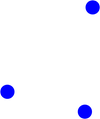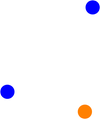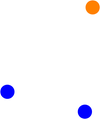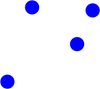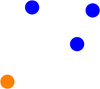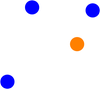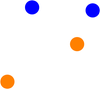对于$\,\mathcal X\,$中的$\,N\,$个点$\,\bm x_1,\bm x_2,\cdots,\bm x_N$，我们称$$\mathcal H(\bm x_1,\bm x_2,\cdots,\bm x_N)=\{(h(\bm x_1),h(\bm x_2),\cdots,h(x_N))|h\in\mathcal H\}$$为$\,\mathcal H\,$对这些点的一个对分．然后我们再引入成长函数$$m_{\mathcal H}(N)=\max_{\bm x_1,\cdots,\bm x_N\in\mathcal X}|\mathcal H(\bm x_1,\bm x_2,\cdots,\bm x_N)|.$$它就表示对于有限个点，$\mathcal H\,$中有效函数的个数．注意到前面的例子中，如果平面上有三点共线的话，直线的种类会更少，所以要取最大值．这样，对于前面的例子，就有$$m_{\mathcal H}(1)=2,m_{\mathcal H}(2)=4,m_{\mathcal H}(3)=8,m_{\mathcal H}(4)=14.$$现在，我们就可以把\eqref{eq10091}式改写为\begin{equation}\label{eq10092}P[\mathcal D \text{ is bad}]\leqslant2m_{\mathcal H}(N)\mathrm e^{-2\varepsilon^2N}.\end{equation}此外，不难看到，对于二分类问题总有$$m_{\mathcal H}(N)\leqslant 2^N.$$

对于数轴上的$\,N\,$个点$\,x_1,x_2,\cdots,x_n$，当$\,\mathcal H=\{\sgn(x-a)|a\in\mathbb R\}\,$时，容易计算$$m_{\mathcal H}(N)=N+1;$$当$\,\mathcal H=\{\sgn((x-a)(x-b))|a<b;a,b\in\mathbb R\}\,$时，容易计算$$m_{\mathcal H}(N)=\binom{N+1}2+1=\frac{N^2}2+\dfrac N2+1.$$当$\,\bm x_1,\bm x_2,\cdots,\bm x_N\,$是平面中同一个圆周上的点时，取$$\mathcal H=\left\{\left.h(\bm x)=\begin{cases}1,&\bm x\in\mathcal C\\-1,&\bm x\not\in\mathcal C\end{cases}\right|\mathcal C\subset\mathbb R^2\text{ is convex}\right\},$$那么容易计算$$m_{\mathcal H}(N)=2^N.$$此时这$\,N\,$个点所有可能的分类情况都属于$\,\mathcal H$，我们说这$\,N\,$个点被$\,\mathcal H\,$打碎．

于是我们看到，当用区间对数轴上的点进行分类时，成长函数是多项式型的，此时\eqref{eq10092}式的右边可以容易地取到较小值，机器学习仍是可行的；当用凸集对圆周上的点进行分类时，成长函数是指数型的，这不是理想的结果，机器学习可能不可行．

我们把满足$\,m_{\mathcal H}(k)<2^k\,$的最小的$\,k\,$叫做$\,m_{\mathcal H}(N)\,$的转折点．于是从前面的例子我们马上知道：用直线对平面上的点进行分类时，转折点是$\,4$；用两个区间对数轴上的点进行分类时，转折点是$\,2$；用三个区间对数轴上的点进行分类时，转折点是$\,3$；用凸集对圆周上的点进行分类时，没有转折点．

如果没有转折点，很显然$\,m_{\mathcal H}(N)\equiv 2^N$；如果有转折点$\,k$，由用区间对数轴进行分类的例子，我们可以猜测$\,m_{\mathcal H}(N)=O(N^{k-1})$．对于这一猜想的证明或证伪，我们以后再讨论．

参考资料 台湾大学《机器学习基石》